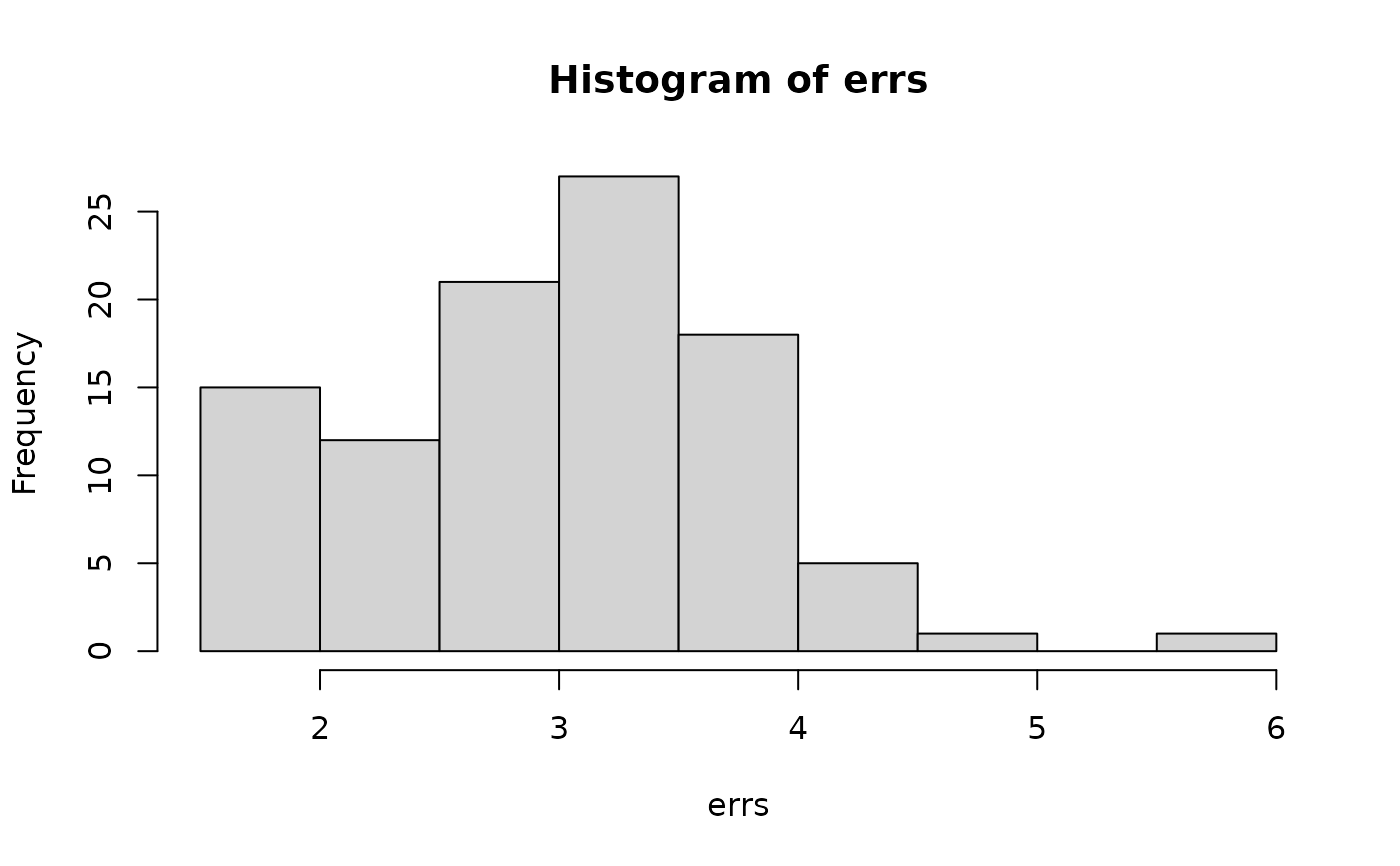crossv_kfold splits the data into k exclusive partitions, and uses each partition for a test-training split. crossv_mc generates n random partitions, holding out test of the data for training. crossv_loo performs leave-one-out cross-validation, i.e., n = nrow(data) training partitions containing n - 1 rows each.

## Usage

crossv_mc(data, n, test = 0.2, id = ".id")

crossv_kfold(data, k = 5, id = ".id")

crossv_loo(data, id = ".id")

## Arguments

data

A data frame

n

Number of test-training pairs to generate (an integer).

test

Proportion of observations that should be held out for testing (a double).

id

Name of variable that gives each model a unique integer id.

k

Number of folds (an integer).

## Value

A data frame with columns test, train, and .id. test and train are list-columns containing resample() objects. The number of rows is n for crossv_mc(), k for crossv_kfold()

and nrow(data) for crossv_loo().

## Examples

cv1 <- crossv_kfold(mtcars, 5)
cv1
#> # A tibble: 5 × 3
#>   train                test                .id
#>   <named list>         <named list>        <chr>
#> 1 <resample [25 x 11]> <resample [7 x 11]> 1
#> 2 <resample [25 x 11]> <resample [7 x 11]> 2
#> 3 <resample [26 x 11]> <resample [6 x 11]> 3
#> 4 <resample [26 x 11]> <resample [6 x 11]> 4
#> 5 <resample [26 x 11]> <resample [6 x 11]> 5

library(purrr)
cv2 <- crossv_mc(mtcars, 100)
models <- map(cv2$train, ~ lm(mpg ~ wt, data = .)) errs <- map2_dbl(models, cv2$test, rmse)
hist(errs)